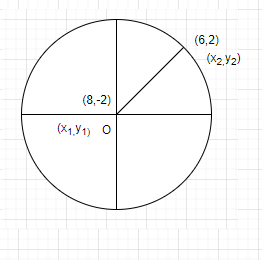QUESTION

# The radius of the circle passing through the point (6,2) and two of whose diameters are $x + y = 6$ and $x + 2y = 4$ is:$A{\text{ 4}} \\ {\text{B 6}} \\ {\text{C 20}} \\ {\text{D}}\sqrt {20} \\$

Hint – Here we will proceed by solving the equations of diameter to get the centre of the circle. Also we will use the distance formula i.e. $d = \sqrt {{{\left( {{x_2} - {x_1}} \right)}^2} + {{\left( {{y_2} - {y_1}} \right)}^2}}$ to get the right radius.The diameter of a circle always passes through its centre.
From the above statement we will find the centre of the circle by solving the equations of diameter simultaneously.
Now we are given with equations of the diameters are
$\Rightarrow x + y = 6$………….. (1)
$\Rightarrow x + 2y = 4$ …………………………………. (2)
Solving equations by substitution method,
Taking equation 1 i.e. $x + y = 6$
Converting it into equation 3,
i.e. $y = \dfrac{{4 - x}}{2}$
Now we will substitute the value of equation 3 in equation 1,
$\Rightarrow x + \dfrac{{4 - x}}{2} = 6$
$\Rightarrow 4 - x = \left( {6 - x} \right)2$
$\Rightarrow 4 - x = 12 - 2x$
$\Rightarrow - 8 = - x$
Or x=8
Now we will substitute value of x=8 in equation 1 i.e. $x + y = 6$ ,
$\Rightarrow 8 + y = 6$
Or y = -2
Hence,
x = 8 and y = -2
Therefore, the centre of circle is (8, -2)
We know that radius is the distance from centre to its perimeter.
Also it is given that the circle passes through (6, 2).
Therefore, radius is the distance between these two points using distance formula-
Distance formula- $d = \sqrt {{{\left( {{x_2} - {x_1}} \right)}^2} + {{\left( {{y_2} - {y_1}} \right)}^2}}$
Where ${x_1} = 8,{x_2} = 6 \\ {y_1} = - 2,{y_2} = 2 \\$
Radius = $\sqrt {{{\left( {6 - 8} \right)}^2} + {{\left( {2 - \left( { - 2} \right)} \right)}^2}}$
$\Rightarrow \sqrt {20}$
Hence option D is right.
Note – We can also use elimination method or substitution method to solve the above mentioned equations. As here we used one of the properties of a circle i.e radius is distance from centre to its perimeter then only we are able to know that we should use distance formula, therefore we must know all the properties of the circle.